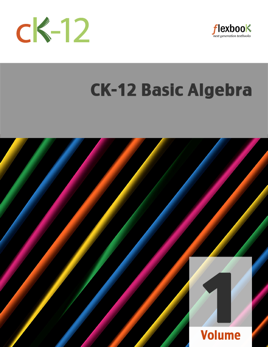## Publisher Description

CK-12 Foundation's Basic Algebra, Volume 1 FlexBook covers the following six chapters:

Expressions, Equations, and Functions - covers the relationships among expressions, equations, and functions when variables are present. Also explored is how these ideas can be shown on graphs. Properties of Real Numbers - covers various forms that rational numbers can assume, including fractions, integers, and square roots. Also considered are different operations for manipulating rational numbers. Linear Equations - introduces students to methods of solving simple equations involving variables. Also covered are ratios, scale, and the percent equation. Graphing Linear Equations and Functions - provides students with a more in-depth understanding of equations by introducing coordinate plane graphing concepts such as intercepts and slope. Writing Linear Equations - focuses on writing various forms of equations based upon real-world data and already existing lines. Students will also learn about predicting data using a fitted line. Linear Inequalities and Absolute Value; An Introduction to Probability - covers operations of inequalities, including addition, subtraction, multiplication and division. These principles are then applied to absolute value and probability.

## Customer Reviews

Pasphill ,

### Basic

Works ok, a little slow at opening, covers basic really well

Tomcatcool ,

### dusnt deserve -1000000

dusnt even open! 😔